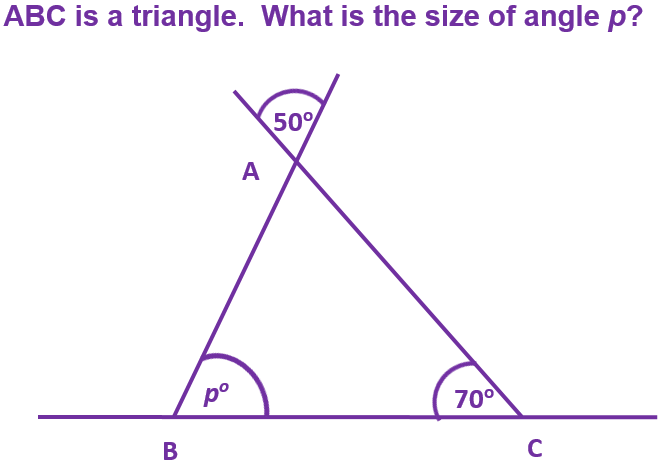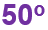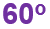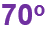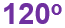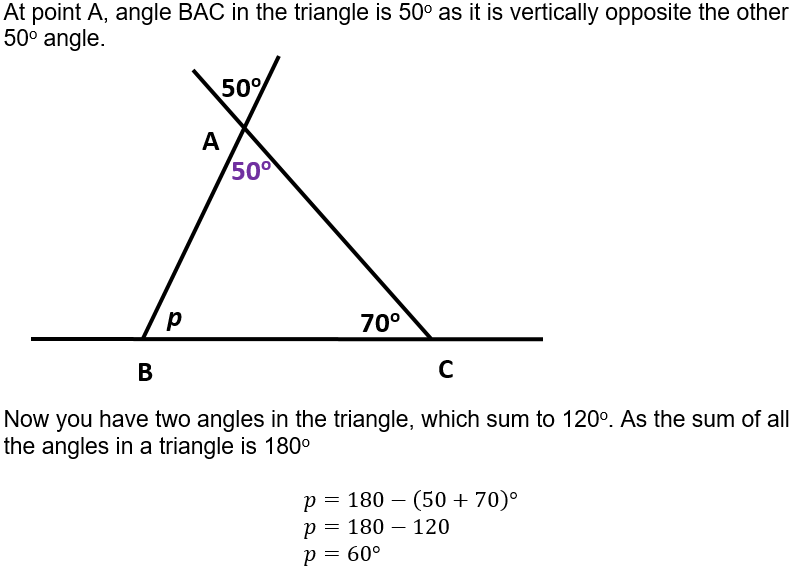To be successful, a snooker player needs to know all about angles.

# Angles (F)

This GCSE Maths quiz will test your knowledge of key angle facts. Angles are everywhere! Look around you, how many can you see?

An angle is formed when two lines meet each other at a point, or cross each other (the correct term for this is INTERSECT). Armed with a few basic angle facts, you should be able to work out all the missing angles in a drawing.

We all remember that there are 360o in a circle, but have you ever wondered why? One theory is that is goes back to the time of the ancient Babylonians, who thought it took 360 days for the Earth to go round the Sun. We now know it takes a little bit longer, but aren’t you glad they didn’t change the number of degrees in a circle to 365o

All our angle facts are developed from there being 360o in a circle. Thinking about it slightly differently, it means all the angles around a point will add up to 360o.

From this, we can work out that angles on a straight line will add up to 180o. Halving that again gives us 90o, known as a right angle. This angle is so important that it even gets its own special symbol, a square. In a drawing, never assume an angle is 90o unless the square is drawn in too.

It’s a good idea to arm yourself with the alphabet when dealing with angles – specifically you need C, F, X and Z. You also need to know the proper name for these types of angles – co-interior, corresponding, vertically opposite and alternate. C, F and Z angles occur where two or more parallel lines are crossed by another line.

Question 1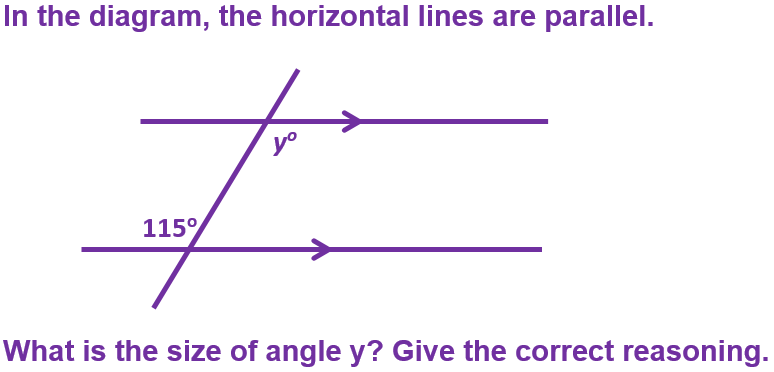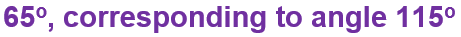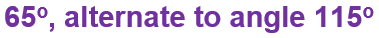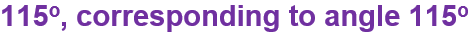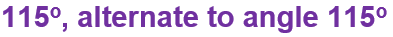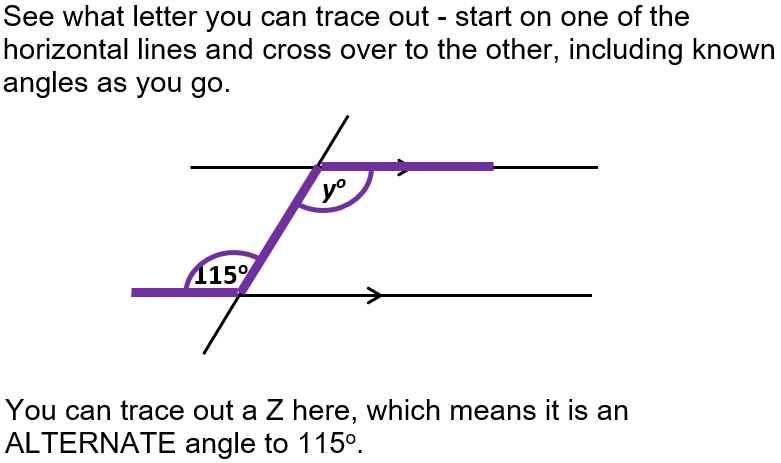Question 2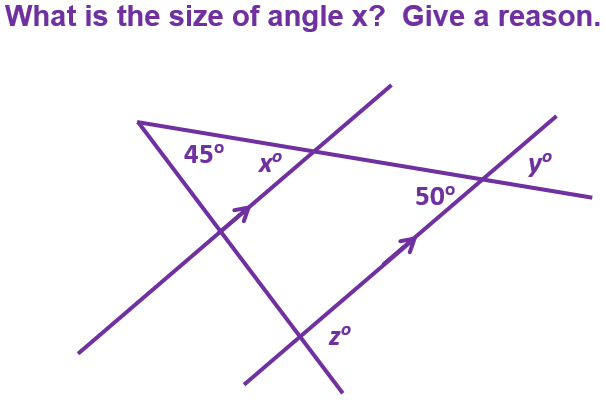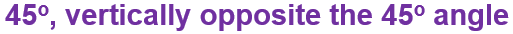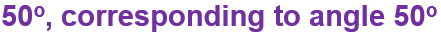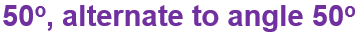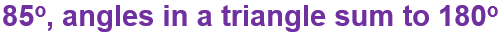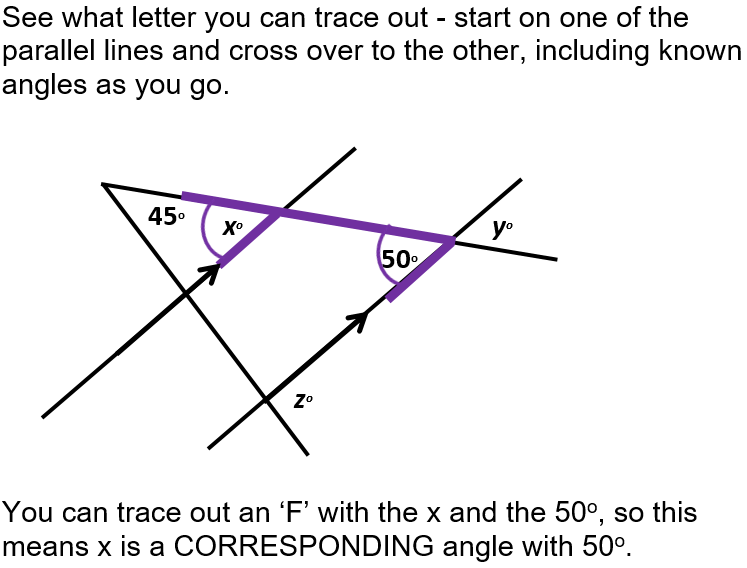Question 3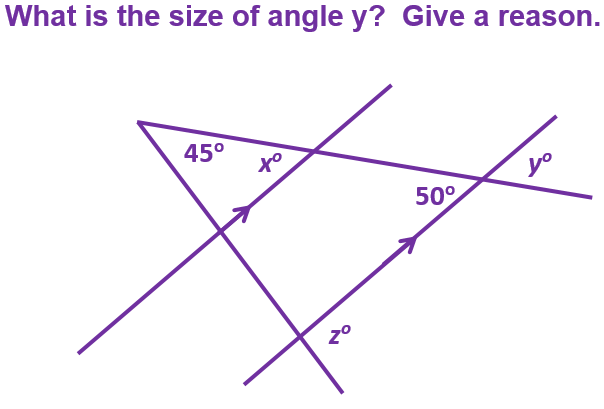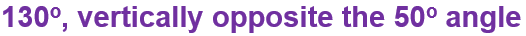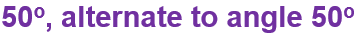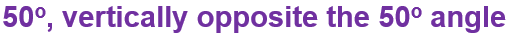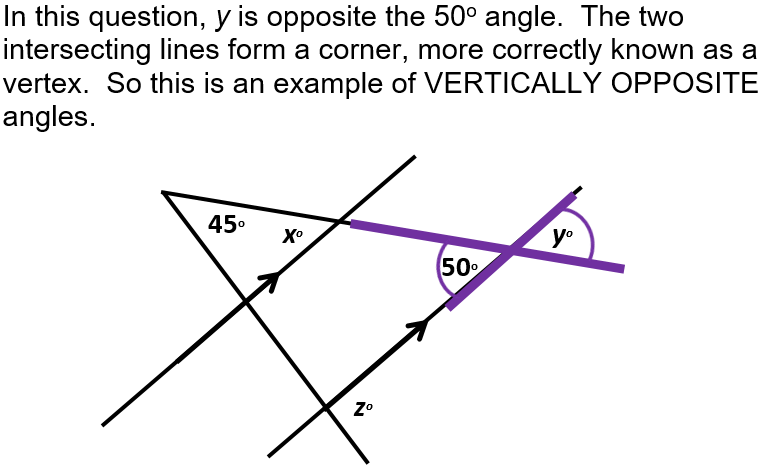Question 4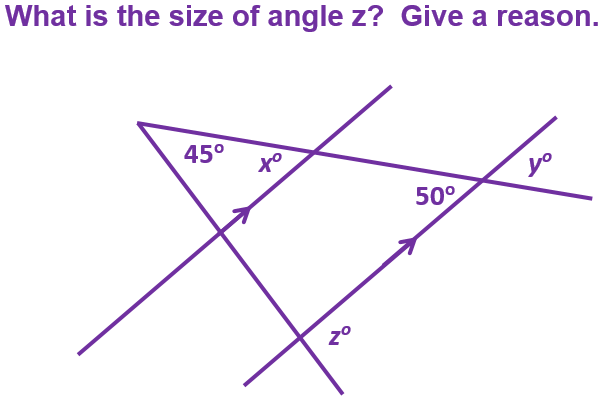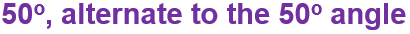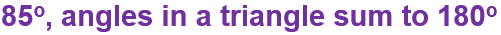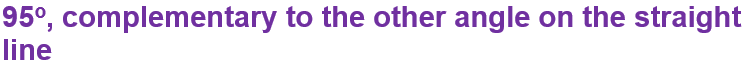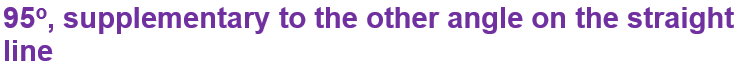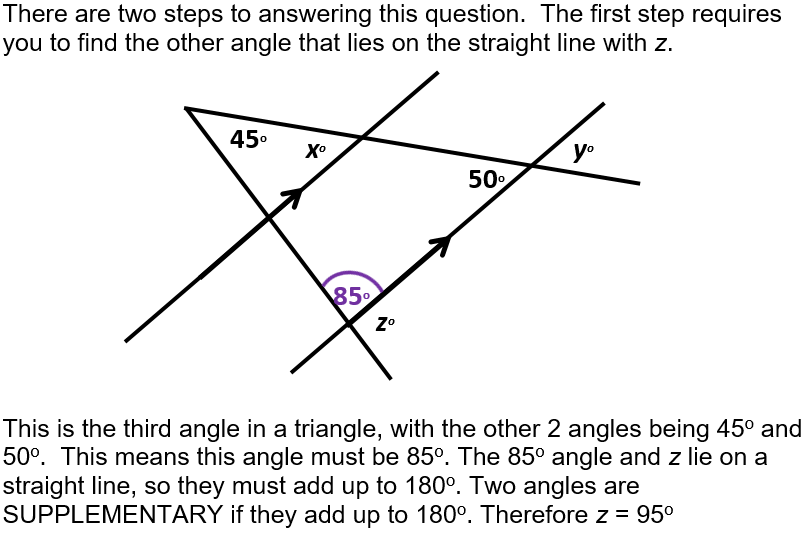Question 5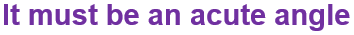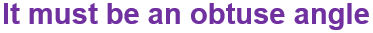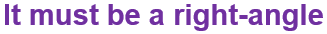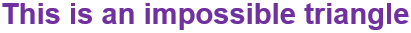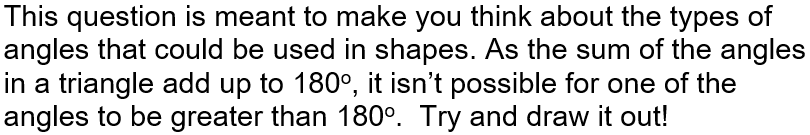Question 6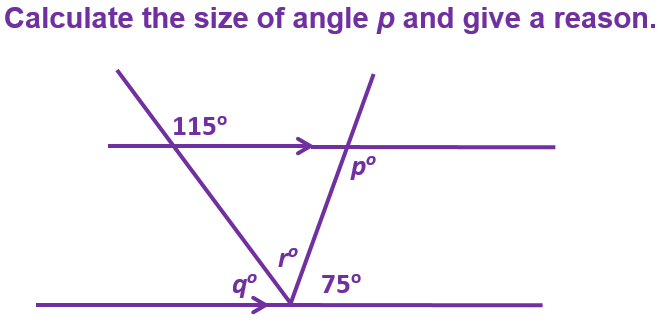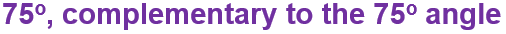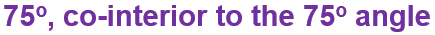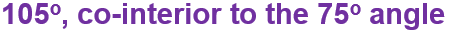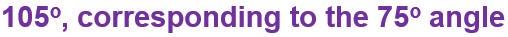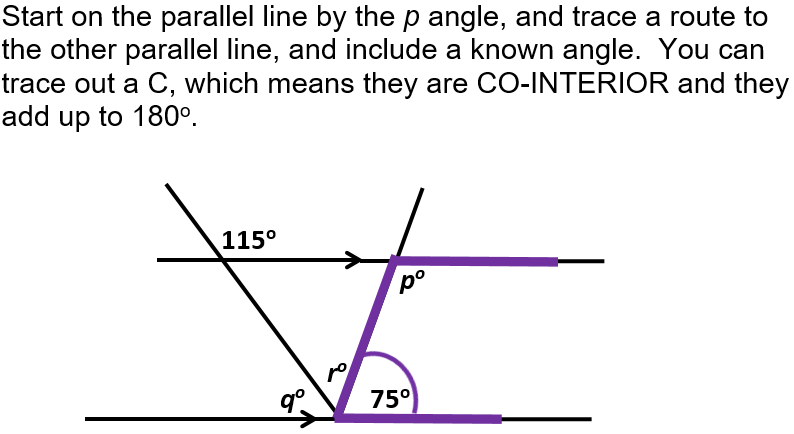Question 7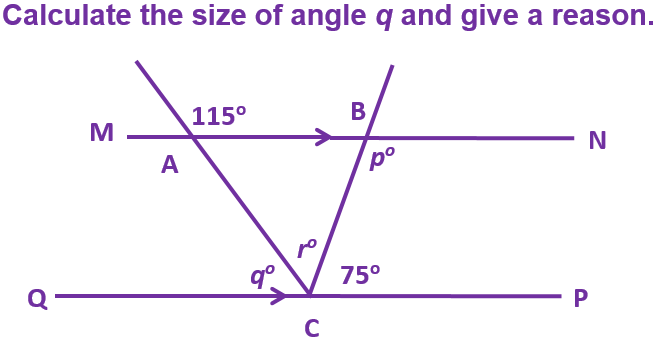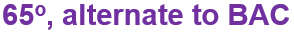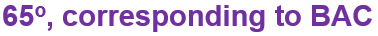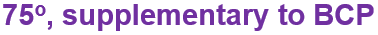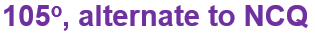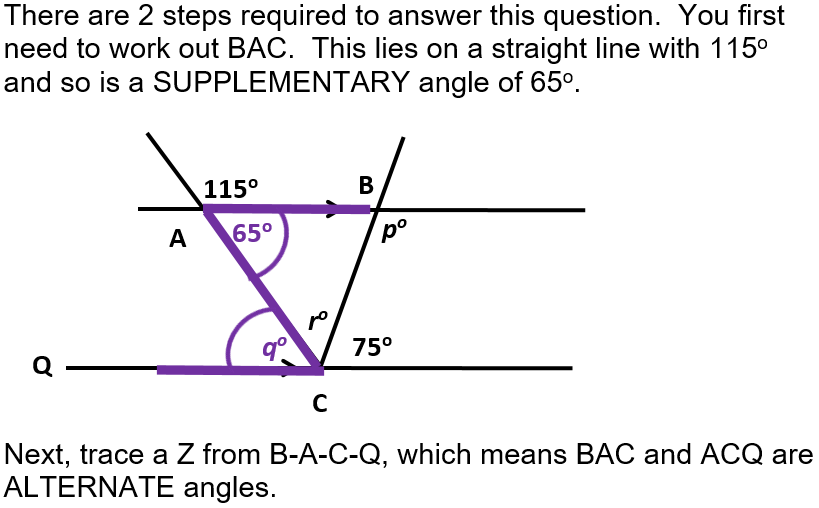Question 8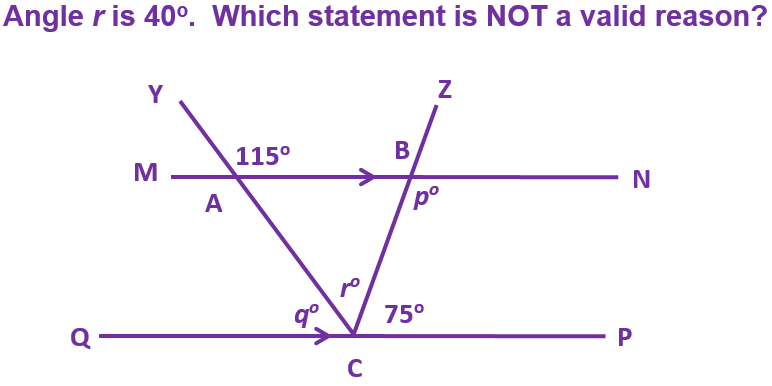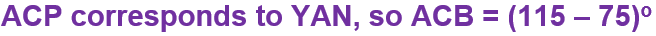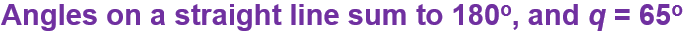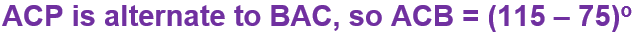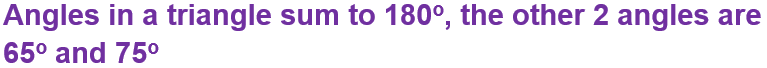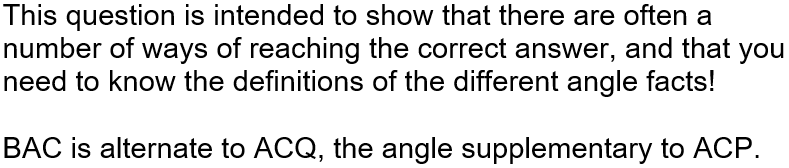Question 9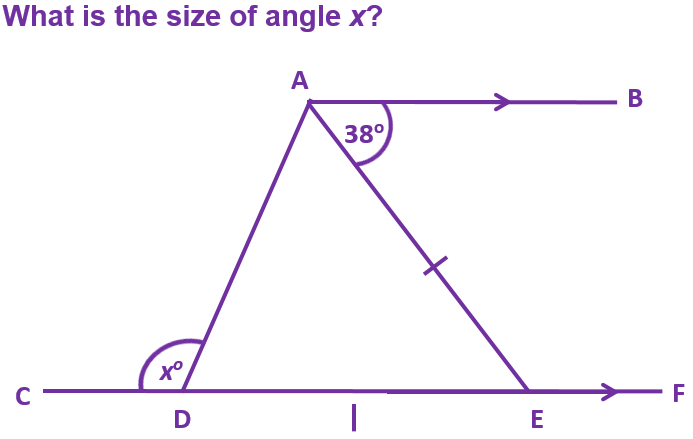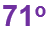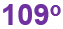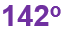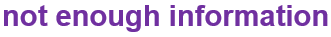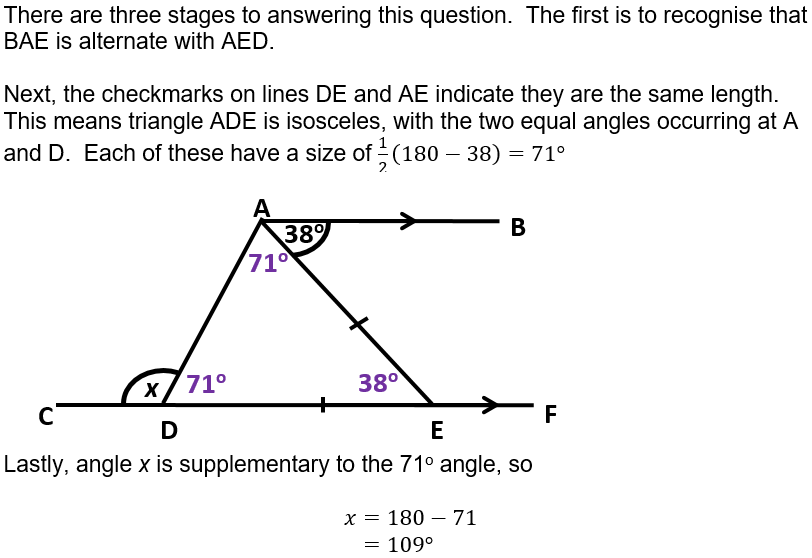Question 10